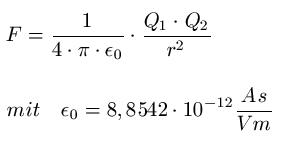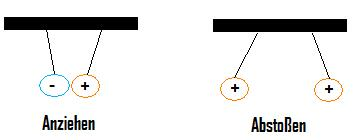# What is Coulomb's law

## Coulomb's law

Coulomb's law is discussed in this article. We will explain to you what is meant by Coulomb's law and provide you with the appropriate formula. This article belongs to the field of physics or electrical engineering.In order to understand Coulomb's law at all, one must know the meaning of the terms charge and coulomb. If these two terms don't mean anything to you, please read them first in the following article:

Show:

### Coulomb's law

Coulomb's law is a very important law from the fundamentals of Electrostatics. Expressed in words, it means: The force between two point charges is directly proportional to the product of the two charges and inversely proportional to the square of their distance. The direction of the force coincides with the line connecting the two charges. So and if you haven't understood that, just put the appropriate values ​​in the following equation:Where:

• "F" is the force in Newtons
• "Q1"and" Q2"the charges in coulombs
• "r" is the distance in meters
• 0"the electric field constant
• "π" the circle number, π = 3.14159 ...

The numerical value for the electric field constant cannot be determined theoretically, but must be measured in an experiment. The force between the charges is repulsive when the charges have the same sign. This results in F> 0. If the sign of the two charges is different, the two charges attract each other, the force F is less than zero (F <0).Coulomb's law makes use of the notion of a Point charge. Imagine a charge that is only present at a single point. However, this is a theoretical model concept. In reality it is the case that a charge can never sit in one point, but always only on a body with finite dimensions. Culomb's law can still be used in practice: If the charges are evenly distributed on a spherical surface, the distance between the center of the sphere can be understood as the distance between corresponding point charges.

Left:

### Who's Online

We have 400 guests online